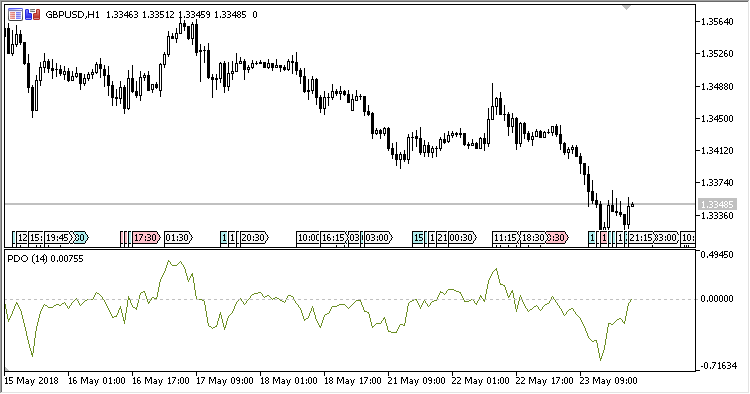# PDO – indicator for MetaTrader 5

PDO (Percent Difference Oscillator) oscillator shows the difference between moving average and the current Close price, expressing the difference in the percents of the moving average (above / below the MA).

It has three input parameters:

• Period – MA calculation period;
• Method – MA calculation method;
• Applied price – MA calculation price.
Related Posts

Calculations:

`PDO = 100*(Close - MA)/MA`